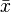# Blog Archives

## MODUS

Mode of a probability distribution

The mode of a discrete probability distribution is the value x at which its probability mass function takes its maximum value. In other words, it is the value that is most likely to be sampled.

The mode of a continuous probability distribution is the value x at which its probability density function attains its maximum value, so, informally speaking, the mode is at the peak.

As noted above, the mode is not necessarily unique, since the probability mass function or probability density function may achieve its maximum value at several points x1, x2, etc.

The above definition tells us that only global maxima are modes. Slightly confusingly, when a probability density function has multiple local maxima it is common to refer to all of the local maxima as modes of the distribution. Such a continuous distribution is called multimodal (as opposed to unimodal).

In symmetric unimodal distributions, such as the normal (or Gaussian) distribution (the distribution whose density function, when graphed, gives the famous “bell curve”), the mean (if defined), median and mode all coincide. For samples, if it is known that they are drawn from a symmetric distribution, the sample mean can be used as an estimate of the population mode.

## MEAN

In statistics, mean has two related meanings:

• the arithmetic mean (and is distinguished from the geometric mean or harmonic mean).
• the expected value of a random variable, which is also called the population mean.

There are other statistical measures that use samples that some people confuse with averages – including ‘median’ and ‘mode’. Other simple statistical analyses use measures of spread, such as range, interquartile range, or standard deviation. For a real-valued random variable X, the mean is the expectation of X. Note that not every probability distribution has a defined mean (or variance); see the Cauchy distribution for an example.

For a data set, the mean is the sum of the values divided by the number of values. The mean of a set of numbers x1, x2, …, xn is typically denoted by, pronounced “x bar”. This mean is a type of arithmetic mean. If the data set were based on a series of observations obtained by sampling a statistical population, this mean is termed the “sample mean” () to distinguish it from the “population mean” (μ or μx). The mean is often quoted along with the standard deviation: the mean describes the central location of the data, and the standard deviation describes the spread. An alternative measure of dispersion is the mean deviation, equivalent to the average absolute deviation from the mean. It is less sensitive to outliers, but less mathematically tractable.